SAT II Math I : Other Data Analyses

Example Questions

Example Question #1 : Other Data Analyses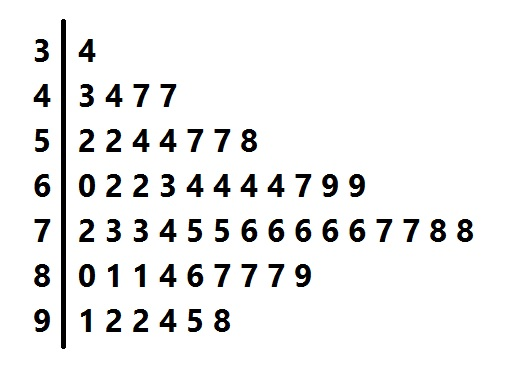Above is the stem-and-leaf display for a group of test scores. Which of the following scores would come closest to being at the 70th percentile?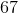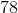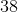Explanation:

The stem-and-leaf display represents 53 scores. The score at the 70th percentile would be the score that is greater than 70% of the 53 scores, or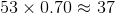scores.

We count up 38 scores from the bottom: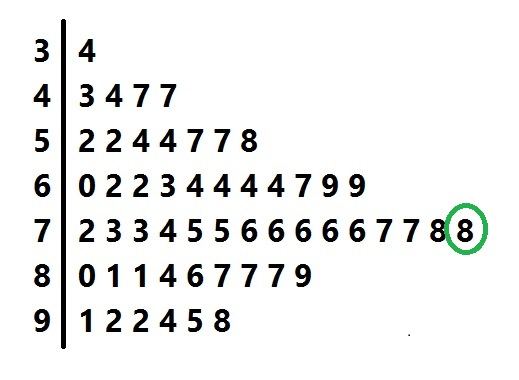The 38th-lowest score is 78, which is the correct choice.

All SAT II Math I Resources